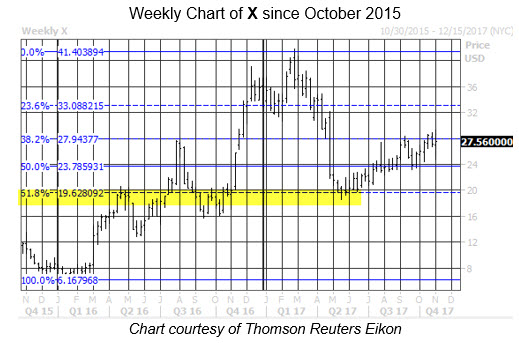How to Use Fibonacci Retracements in Technical Analysis

Stocks tend to "retrace" their paths after a sizable move, regardless of direction

Managing Editor
Nov 3, 2017 at 2:13 PM

Centuries ago, before there was any semblance of a stock market, one Italian developed a theory that would lay the groundwork for countless mathematical applications. Fibonacci retracements are valuable technical analysis tools that enable an investor to sniff out a stock's possible support or resistance areas. Looking at Fibonacci retracements, or "Fib levels,"as we call them, can give investors a leg up in their stock analysis. Below, we will breakdown what a Fibonacci retracement level is, and discuss the relationship to stocks.

Leonardo Fibonacci was a 12th century Italian mathematician who was widely regarded as "the most talented Western mathematician of the Middle Ages." His most famous work is the Fibonacci sequence, where every number after the first two is the sum of the two preceding numbers. Consider the example below:

1, 1, 2, 3, 5, 8, 13, 21, 34, 55, 89, 144, 233, 377, 600 and so on.

This seemingly innocuous sequence was the forefront to countless algorithms and formulas that would impact everything from biology to computer science. But what does that short history lesson have to do with stocks and technical analysis? The Fibonacci sequence helps gauge price movement. More specifically, it can be used to forecast equity corrections or pullbacks.

Fibonacci retracement levels operate as percentages based on the collective belief that stocks tend to "retrace" their paths after sizable moves, regardless of direction. A trendline is established between the highs and lows, and then divides the vertical distance by these key Fibonacci ratios: 23.6%, 38.2%, 50%, 61.8% and 100%. As the price of an equity retraces after a significant move in either direction, it may stall at support/resistance near the Fibonacci lines.

Digging deeper, any given number (after the first few small digits) in the ratio is approximately equal to 1.618 times the preceding number and approximately 0.618 times the following number. In fact, 1.618 -- the "golden ratio" -- can be found all over nature, and a 61.8% Fibonacci retracement is often the most telling for a stock. Let's look at an example.

Back in June, United States Steel Corporation (NYSE:X) bounced off a 61.8% Fib retracement of its 2016 low to its 2017 high. Since then, X has climbed. The steel stock, which is currently up 0.4% to trade at \$27.42, now finds itself testing another key level, as \$28 is roughly home to a 23.6% Fibonacci retracement of the same rally.As you can see, Fibonacci levels are worth monitoring when searching for a potential floor of support during a stock's pullback, or a possible speed bump during a rebound. Combined with other technical analyses, as well as sentiment and fundamental analysis -- what we've christened Expectational Analysis -- traders can get a feel for possible points in which to buy or sell, and take some of the guess-work out of trading.

Begin the New Year With Schaeffer's 7 FREE 2022 Stock Picks!